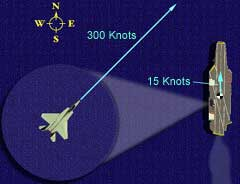Ch 1. Particle General Motion Multimedia Engineering Dynamics Position,Vel & Accel. Accel. varyw/ Time Accel. Constant Rect. Coordinates Norm/Tang. Coordinates Polar Coordinates RelativeMotion
 Chapter - Particle - 1. General Motion 2. Force & Accel. 3. Energy 4. Momentum - Rigid Body - 5. General Motion 6. Force & Accel. 7. Energy 8. Momentum 9. 3-D Motion 10. Vibrations Appendix Basic Math Units Basic Equations Sections Search eBooks Dynamics Fluids Math Mechanics Statics Thermodynamics Author(s): Kurt Gramoll ©Kurt GramollDYNAMICS - CASE STUDY IntroductionThe plane's velocity is relative to the aircraft carrier An F-15's navigation system has been destroyed, and the pilot needs to find his way back to land. He passes the aircraft carrier Nimitz, whose radar operator is able to determine the F-15's velocity relative to the carrier. How can the pilot use this information to find his way home? What is known: The Nimitz is traveling north at 15 knots (nautical miles per hour) relative to the earth. The radar operator on the carrier determines that the velocity of the F-15 relative to the carrier is horizontal and of magnitude 300 knots to the northeast. Question What are the magnitude and direction of the plane's velocity relative to the earth? Approach The carrier's velocity relative to the earth, and the velocity of the plane relative to the carrier are known. By developing equations relating the motion of two or more objects, the motion of the plane relative to the earth based on its motion relative to the carrier can be determined.

Practice Homework and Test problems now available in the 'Eng Dynamics' mobile app
Includes over 400 problems with complete detailed solutions.
Available now at the Google Play Store and Apple App Store.